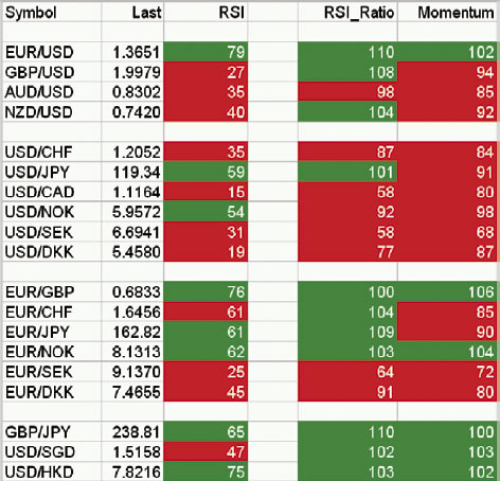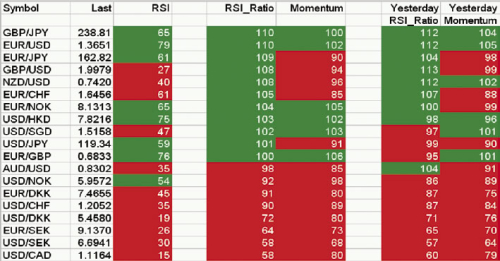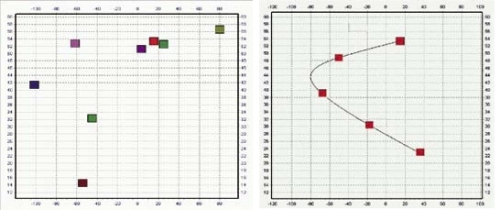ABSTRACT

Most novel forex traders trade only one pair at time trying to make money using indicators, trading systems and money management. In this article I will explain an easy way to stick to the trend in Forex diversifying your risk through a portfolio of currency pairs. In other words, readers will learn to compose portfolios of pairs so that the losses of some pairs should be compensated, at least, or even exceeded, by the benefits of rest.

1 INTRODUCTION

To diversify our risk in Forex we are going to create a "Ranking of pairs" using an homogeneous measure. There are several techniques about how to create a ranking of pairs. In this article I will give a very practical view of a general method that readers can use to compose their own Forex portfolio. This method is illustrated with an example based on the Schelling's approach, which I think that it can be very interesting for this community.

The article starts describing shortly some considerations (concepts and indicators) necessary to understand the example. Then all the steps of the method are detailed through an example using the Schelling's approach. Next, the article discusses a brief strategy based on the created portfolio showing how to exploit it. Finally and before the references, some interesting conclusions are presented.

2 PREVIOUS CONSIDERATIONS

Concepts:
• Diversification: "In finance, diversification means reducing risk by investing in a variety of assets" ().
Indicators
• RSI: The Relative Strength Index (RSI) is a "technical indicator ... intended to chart the current and historical strength or weakness of a stock or market based on the closing prices of a recent trading period." ()
• The Rate of Change (ROC) is a "simple technical analysis indicator showing the difference between today's closing price and the close N days ago" ().
• SMA: A Simple Moving Average (SMA) is "the unweighted mean of the previous n datum points" (). For this article, we consider SMAs of 10 and 30 periods.

Remarks:
• In all the examples we use daily data taking at the closing time in New York (22.00 h. CET).

3 FOREX PORTFOLIO GENERATION. A GENERAL METHOD

3.1 STEP 1. Select an homogeneous measure for all the pairs.

To illustrate the method we follow the Schelling's approach but readers are free to use any other indicator. Schelling uses the RSI (). However, RSI can be very volatile on daily charts so we will soften it using two Simple Moving Averages (SMAs) of 10 and 30 periods respectively. The chart below shows an example applied to the EURUSD:We are going to use those averages to create a ratio of the strength or weakness of the pair. To do this, we divide the SMA of 10 periods of the RSI by the SMA of 30 periods, and we multiply the result by 100, obtaining a smoothed measure of the relative force of the pair. The result is shown in the graph below (RatioMA_RSI at the bottom of the image):3.2 STEP 2. Measuring the momentum.

We already have an indicator of the strength of the trend of a pair. Now we need an indicator to measure the speed or momentum of the movement of the pairs. Following with the Schelling's approach, we will use for our example the ROC indicator (Rate of Change ) but again, readers are free to use any other indicator:3.3 STEP 3. Computing the ranking.

All we need to do now is to export all these values to our favorite spreadsheet editor such as LibreOffice, OpenOffice, or Excel. This will allow us to have an overview of the forex market. In the next image, the red and green colors show:
• If the RSI is below or above 50.
• If the RSI and the ROC are below or above 100.3.4 STEP 4. Create the portfolio.

Now it's time to create the ranking of currencies based on the previous values​​. If we look at the following table, we see that the GBPJPY pair has an average ratio of 110 on RSI and a "time" measured by the ROC of 100, while the previous day's average ratio RSI marked 112 and the time was 104. In any case, by having all its values ​​in green is clear that the pair GBPJPY was more bullish. We then sort all the pairs from the most bullish or "strong" (all values ​​in green) to the most bearish or "weak" (all values ​​in red).4 DESIGN YOUR PORTFOLIO STRATEGY

Now we present a strategy based on this information: buy stronger pairs and sell the weakest, adjusting the positions daily as information is changing in our spreadsheet. Coming back to the example and depending upon the size of your account, each day we will have long positions in the three or four strongest markets and short positions in the three or four weakest pairs in our created portfolio. Remember to adjust the positions when necessary.

6 CONCLUSION

We have shown a general method to create a portfolio of pairs of currencies. The method has been illustrated with an example from the Ron Schelling's article about Ranking Forex Markets, but readers are free to use any other indicators to customize their own portfolio.

In conclusion, what Schelling's approach makes is very close to the "heatmap" concept (out of the scope of this article) and can be applied to other financial markets using other indicators to create portfolios. Note that the Schelling's approach does not use the RSI as an oscillator of overbought / oversold but of the strength or weakness of the pair.

If you want me to upload my JForex implementation (indicator RatioMA_RSI) that exports data to a spreadsheet following the Schelling's approach, feel free to ask me for it in the comments or by private message and I will send it to you with pleasure.

All the images of this article belongs to the original article from the Schelling's approach and are used for educational purposes only in the framework of this contest.

REFERENCES

 Diversification: http://en.wikipedia.org/wiki/Diversification_(finance)
 RSI: http://en.wikipedia.org/wiki/Relative_Strength_Index
 ROC: http://en.wikipedia.org/wiki/Rate_of_change_(technical_analysis)
 Ron Schellingan's article: "Ranking Forex Market" available to the public here.
 SMA: http://en.wikipedia.org/wiki/Moving_average#Simple_moving_average

APPENDIX A

A different representation is a scatter plot of RSI (vertical axis) and ROC (horizontal axis) as shown below (left):In the above chart (left) we have high values ​​of RSI and ROC in the upper right corner, while values ​​appear at the bottom left represent pairs with low values ​​for RSI and ROC.

We can also see the evolution for each pair of RSI and ROC over the last five days to see if the pair is gaining or losing strength (right).

APPENDIX B

This article is the first one of the Practical Forex Portfolio Management Series. They will cover very practical approaches (like this article with only the necessary theory), concise and precise information, and JForex implementations of the concepts.

If you like them, please let me know so I can continue with the series.

Translate to English Show original